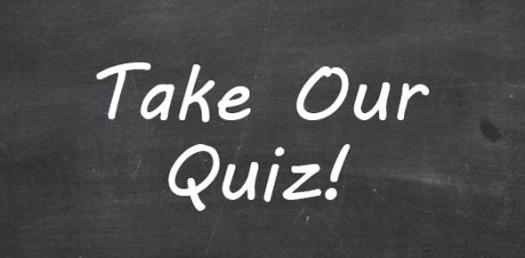# Algebra 1b Checkpoint A Period

25 Questions | Total Attempts: 38SettingsFormative Assessment on Quarter 1 Topics.

• 1.
Solve the equation:
• A.

-1

• B.

7

• C.

-2

• D.

-13

• 2.
Solve the equation:
• A.

1

• B.

-11

• C.

11

• D.

15

• 3.
Solve the equation:
• A.

-15

• B.

10

• C.

-7

• D.

-8

• 4.
Solve the equation:
• A.

-5

• B.

-9

• C.

-3

• D.

7

• 5.
Solve the equation:
• A.

14

• B.

3

• C.

4

• D.

No solution

• 6.
Solve the equation:
• A.

-11

• B.

16

• C.

14

• D.

-2

• 7.
Solve the equation:
• A.

9

• B.

3

• C.

4

• D.

All real numbers

• 8.
Solve for y:
• A.

A

• B.

B

• C.

C

• D.

D

• 9.
Solve for y:
• A.

A

• B.

B

• C.

C

• D.

D

• 10.
Solve the inequality:
• A.

A

• B.

B

• C.

C

• D.

D

• 11.
Solve the compound inequality and graph its solution:
• A.

A

• B.

B

• C.

C

• D.

D

• 12.
Solve the compound inequality and graphs its solution:
• A.

A

• B.

B

• C.

C

• D.

D

• 13.
Evaluate the function:
• A.

-11

• B.

-31

• C.

-39

• D.

37

• 14.
Evaluate the function:
• A.

-1

• B.

5

• C.

8

• D.

-25

• 15.
Perform the indicated operation:
• A.

35

• B.

99

• C.

-3

• D.

-35

• 16.
Perform the indicated operation:
• A.

-15

• B.

13

• C.

-3

• D.

-11

• 17.
Determine whether the following is a function. Mark True if it is a function, false if it is not a function.Divide by 7 and add 13
• A.

True

• B.

False

• 18.
Determine whether the following is a function. Mark True if it is a function, false if it is not a function.​Add 3 and multiply by any number larger than the input.
• A.

True

• B.

False

• 19.
Determine whether the following is a function. Mark True if it is a function, false if it is not a function.​InputOutput20324432
• A.

True

• B.

False

• 20.
Determine whether the following is a function. Mark True if it is a function, false if it is not a function.​InputOutput30425-10410
• A.

True

• B.

False

• 21.
Determine whether the following is a function. Mark True if it is a function, false if it is not a function.​
• A.

True

• B.

False

• 22.
State the domain for the function:
• A.

All real numbers

• B.

All real numbers except -7

• C.

All real numbers greater than -7

• D.

X=-7

• 23.
State the domain for the function:
• A.

All real numbers

• B.

All real numbers except -1

• C.

All real numbers less than -1

• D.

X= -1

• 24.
State the explicit rule for the following function: InputOutput041628310
• A.

F(x) = 2x + 4

• B.

F(x) = 4x + 2

• C.

F(x) = 4x - 2

• D.

F(x)= 2x - 4

• 25.
State the recursive rule for the following function: InputOutput0518211314
• A.

G(x) = g(x - 1) + 3, if g(0) = 5

• B.

G(x) = g(x - 1) + 5

• C.

G(x) = g(x - 1) + 3

• D.

G(x) = g(x - 1) + 5, if g(0) = 3

Related Topics